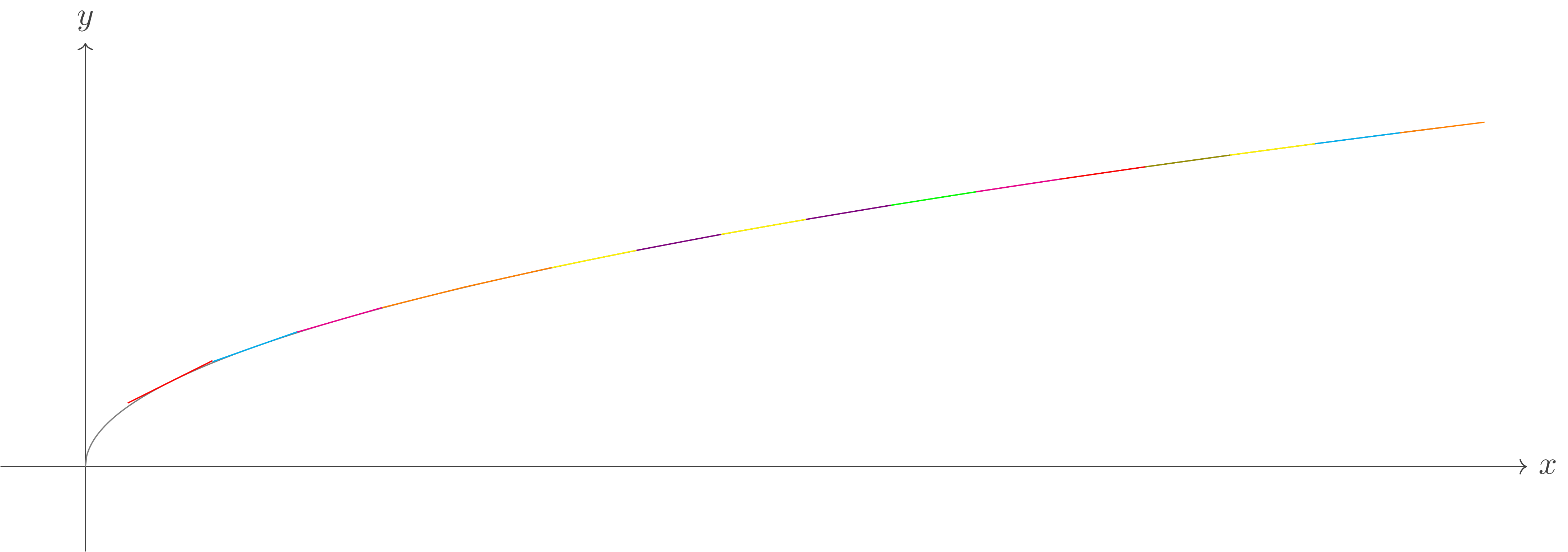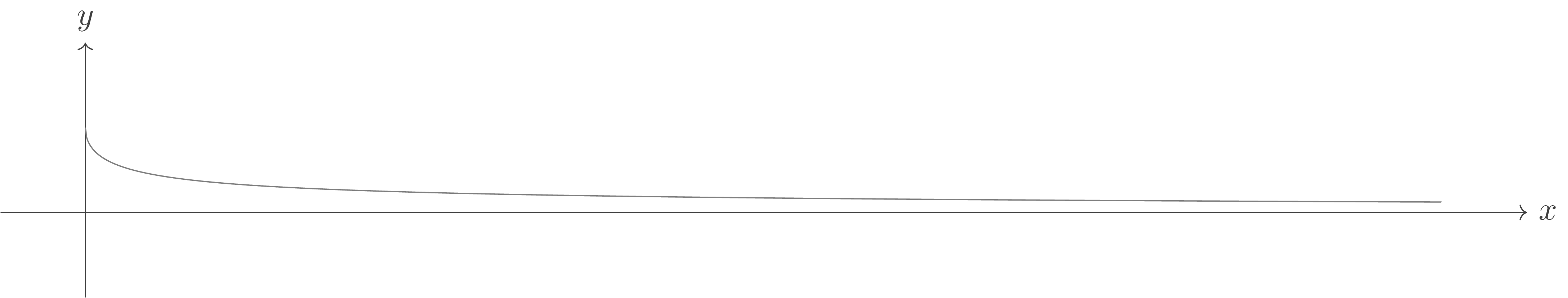# Spacing of Square Roots

The distance between consecutive perfect squares is not uniform:

The size of the space between $n^2$ and $(n+1)^2$ is $2n + 1$, intuitively evidenced by the growth in the spacing of the visualization above.

Now, take a look at the distance between consecutive integer square roots:

In particular, observe the spacing of integer square roots between $4$ and $9$ - at one degree of precision, they are uniformly distributed!

To me, this trend is significantly less intuitive. It makes sense in the "what else is expected?" kind-of way, but that expectation isn't very satisfying. So here are a few other ways of understanding the spacing.

### 1: From the Spacing of Squares

For $n \in \mathbb{N}$, between $n^2$ and $(n+1)^2$ are the natural numbers $n^2+1, n^2+2, \ldots, n^2 + 2n$. So between $n$ and $n+1$, there is a set of integer square root reals $S = \left\{\sqrt{n^2+1}, \sqrt{n^2+2}, \ldots, \sqrt{n^2 + 2n}\right\}$, and $|S| = 2n$. Now, because $S_{i+1} > S_i$, we can make an argumentsomewhat similar to the pigeonhole principle that for $n$ large, there are a large number of integer square roots in the 1-unit interval $(n, n+1)$, giving the perception of uniformity of the monotonically increasing set $S$.

But this explanation is not rigorous, and leaves one hungry for a more elegant explanation.

### 2: From Tangents

Take a look at $f(x) = \sqrt{x}$:$f(x) = \sqrt{x}$ and its tangents, $\frac{d}{dx} f(x)$ on $x\pm 0.5, x\in[0,16]$

Observe that for $n$ large, the slopes of the tangents of $f(x)$ at $n$ and $n+1$ are small and very similar, implying

1. that there is little variation in the value of the function at $n$ and $n+1$, and
2. the variation between $n$ and $n+1$ is very similar to the variation between $n+1$ and $n+2$.

And that's enough needed for a beautiful reasoning of the relative uniformity of integer square roots!

The great thing about this kind of reasoning is it also explains the increasing spacing between nodes of an exponential function, like $x^2$ or $e^x$. In both of these cases, the slopes of pointwise tangent lines are increasing, corresponding to the increasing rate of change in the spacing between e.g. perfect squares.

#### 3: A remix

To understand the previous reasoning a different way, consider $f(x) = \sqrt{x + 1} - \sqrt{x}$.$f(x) = \sqrt{x + 1} - \sqrt{x}$

The difference between consecutive integer square roots decreases asymptotically, and thus we have that there is a relative uniformity in their spacing -- especially at large integer values.

## An Application

A friend asked what practical purpose knowing the spacing of integer square roots serves. One useful application is in square root approximation - knowing that integer square roots are approximately uniform especially at large numbers, we can say that for an integer $q$, $\sqrt{q} \approx n + \frac{q-n^2}{2n+1}$, where $n = {\lfloor{\sqrt{q}}\rfloor}$For example, $10.724 = \sqrt{115} \approx 10.714 = \sqrt{100} + \frac{15}{21}$..

Analytics By visiting this site, you agree to its use of Cloudflare Analytics. No identifiable information is transmitted to Cloudflare. See Cloudflare Analytics user privacy.